人工智能——K-Means聚类算法及Python实现

目录
• 1 概述
• 1.1 无监督学习
• 1.2 聚类
• 1.3 K-Mean均值算法
• 2 K-Mean均值算法
• 2.1 引入
• 2.2 针对大样本集的改进算法：Mini Batch K-Means
• 2.3 图像
• 3 案例1
• 3.1 代码
• 3.2 结果
• 4 案例2
• 4.1 案例——数据
• 4.2 代码
• 4.3 结果
• 4.4 拓展&&改进

1 概述

1.1 无监督学习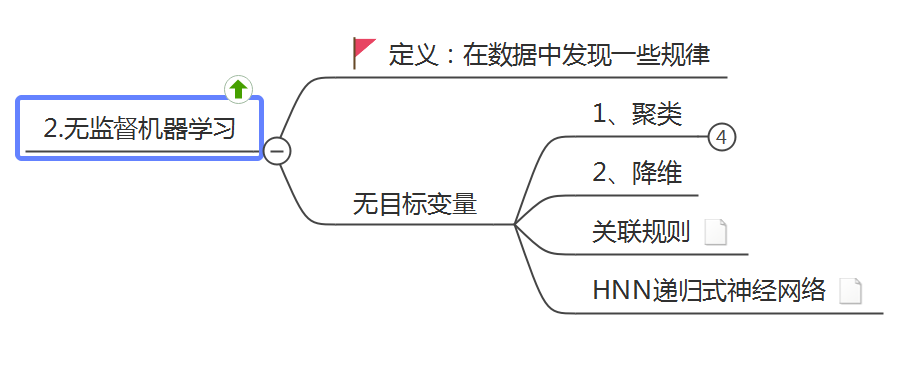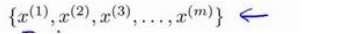这将是我们介绍的第一个非监督学习算法。当然，此后我们还将提到其他类型的非监督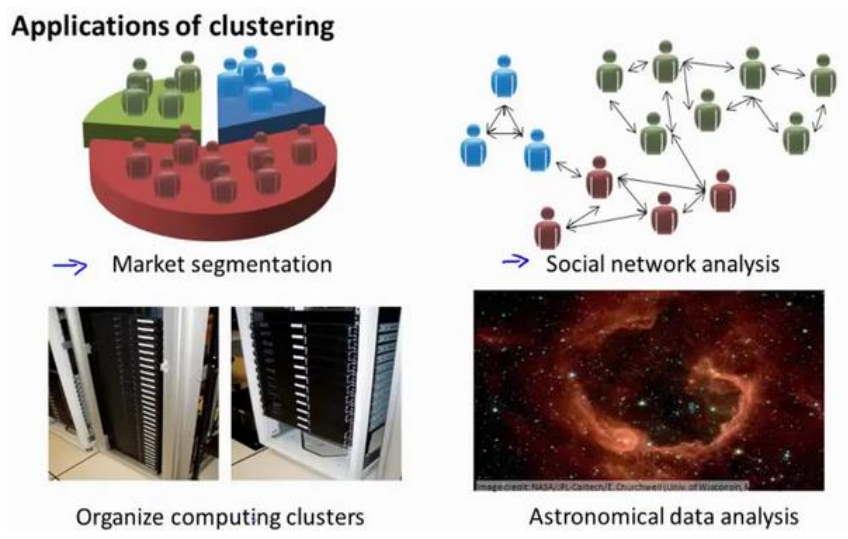最后，我实际上还在研究如何利用聚类算法了解星系的形成。然后用这个知识，了解一

1.2 聚类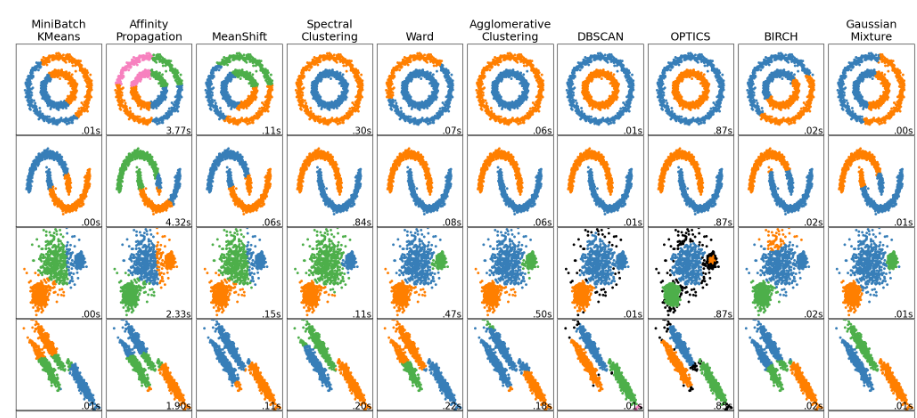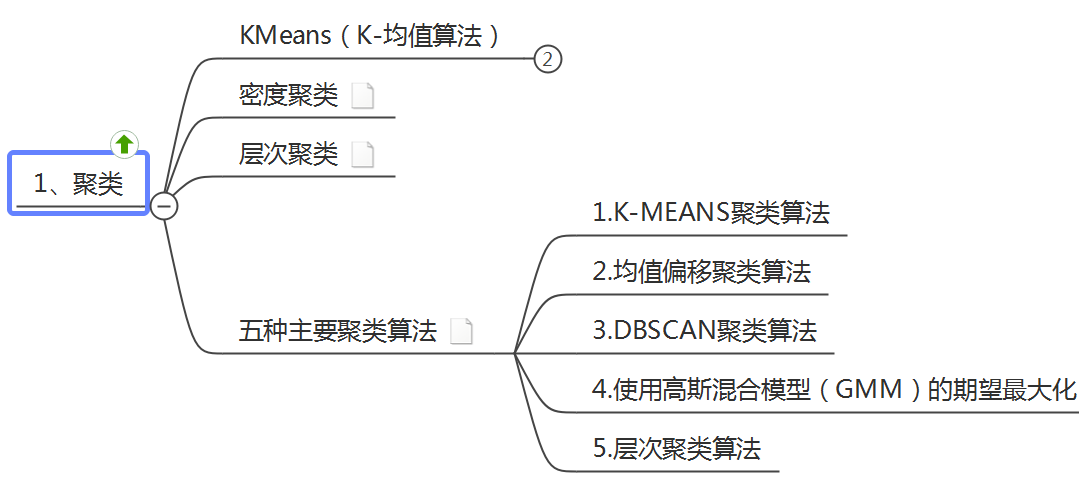1.3 K-Mean均值算法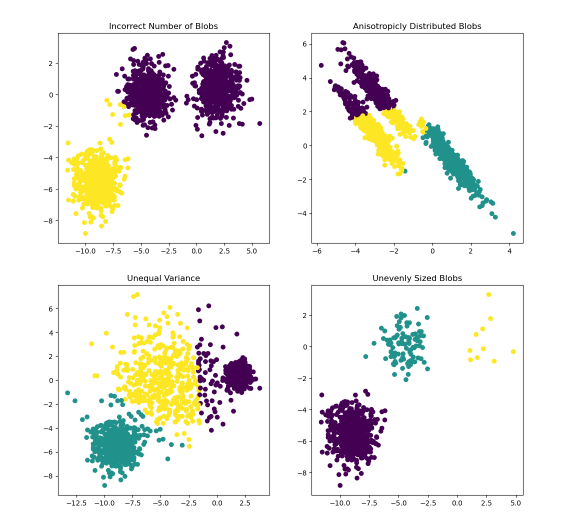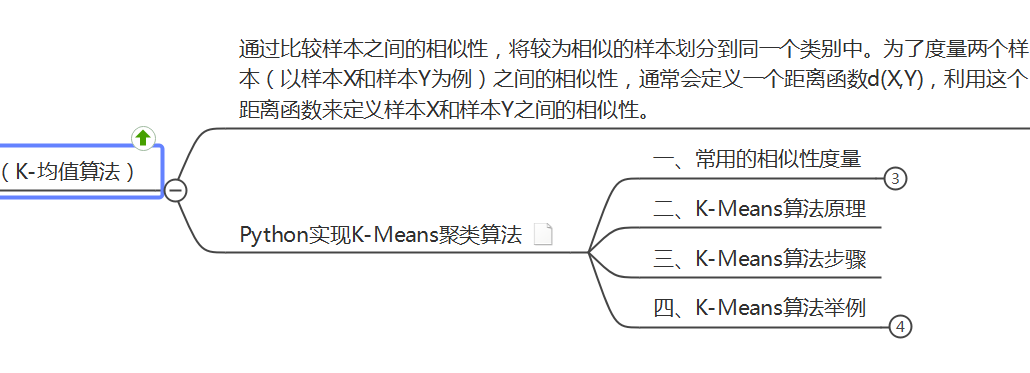2 K-Mean均值算法

2.1 引入

K- 均值 是最普及的聚类算法，算法接受一个未标记的数据集，然后将数据聚类成不同的

• 设定 K 个类别的中心的初值；
• 计算每个样本到 K个中心的距离，按最近距离进行分类；
• 以每个类别中样本的均值，更新该类别的中心；
• 重复迭代以上步骤，直到达到终止条件（迭代次数、最小平方误差、簇中心点变化率）。

K-means聚类算法：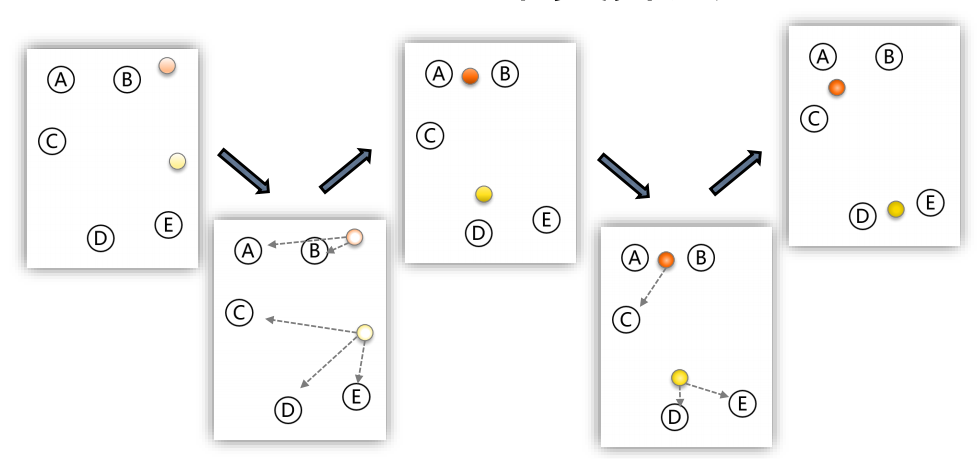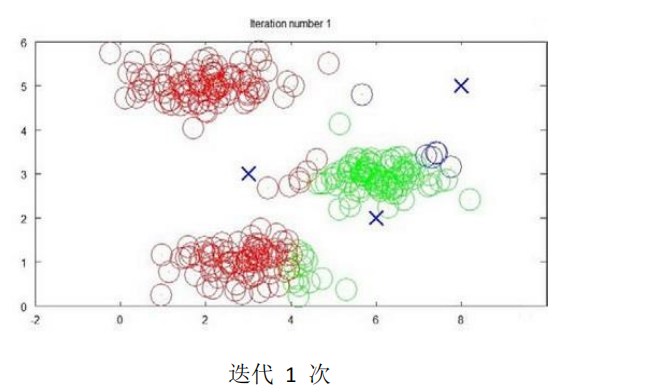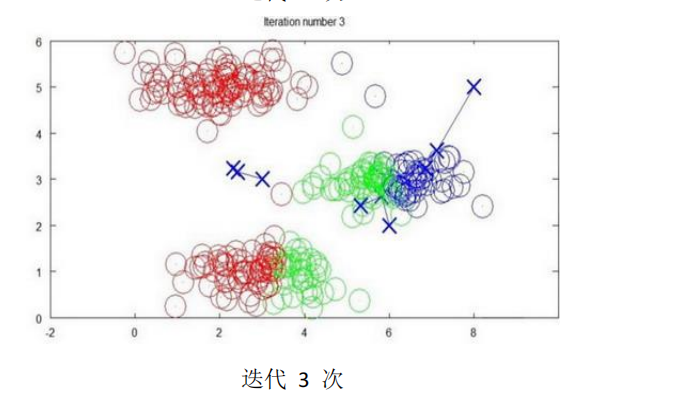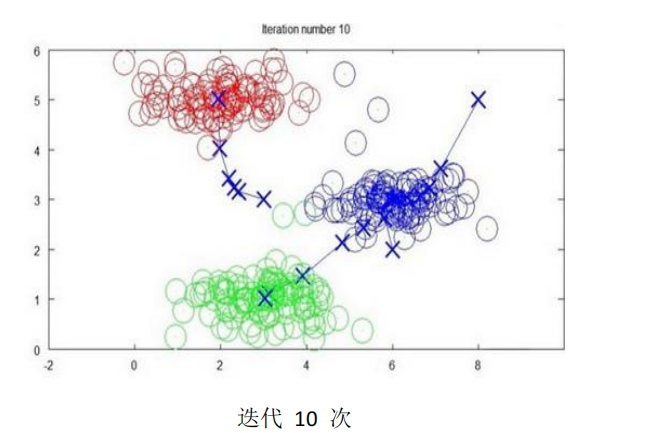K-均值算法的伪代码如下：

Repeat {
for i = 1 to m
c(i) := index (form 1 to K) of cluster centroid closest to x(i)
for k = 1 to K
μk := average (mean) of points assigned to cluster k
}

from sklearn.cluster import KMeans  # 导入 sklearn.cluster.KMeans 类
import numpy as np

X = np.array([[1,2], [1,4], [1,0], [10,2], [10,4], [10,0]])
kmCluster = KMeans(n_clusters=2).fit(X)  # 建立模型并进行聚类，设定 K=2
print("聚类中心坐标:",kmCluster.cluster_centers_)  # 返回每个聚类中心的坐标
print("分类结果:",kmCluster.labels_)  # 返回样本集的分类结果
print("显示预测判断:",kmCluster.predict([[0, 0], [12, 3]]))  # 根据模型聚类结果进行预测判断

[ 1.  2.]]

Process finished with exit code 0

2.2 针对大样本集的改进算法：Mini Batch K-Means

from sklearn.cluster import MiniBatchKMeans # 导入 .MiniBatchKMeans 类
import numpy as np
X = np.array([[1,2], [1,4], [1,0], [4,2], [4,0], [4,4],
[4,5], [0,1], [2,2],[3,2], [5,5], [1,-1]])
# fit on the whole data
mbkmCluster = MiniBatchKMeans(n_clusters=3,batch_size=6,max_iter=10).fit(X)
print("聚类中心的坐标:",mbkmCluster.cluster_centers_) # 返回每个聚类中心的坐标
print("样本集的分类结果:",mbkmCluster.labels_)  # 返回样本集的分类结果
print("显示判断结果：样本属于哪个类别:",mbkmCluster.predict([[0,0], [4,5]]))  # 根据模型聚类结果进行预测判断

[ 0.75862069 -0.20689655]
[ 4.20588235  4.5       ]]

Process finished with exit code 0

2.3 图像

from sklearn.cluster import kmeans_plusplus
from sklearn.datasets import make_blobs
import matplotlib.pyplot as plt

# Generate sample data
n_samples = 4000
n_components = 4

X, y_true = make_blobs(
n_samples=n_samples, centers=n_components, cluster_std=0.60, random_state=0
)
X = X[:, ::-1]

# Calculate seeds from kmeans++
centers_init, indices = kmeans_plusplus(X, n_clusters=4, random_state=0)

# Plot init seeds along side sample data
plt.figure(1)
colors = ["#4EACC5", "#FF9C34", "#4E9A06", "m"]

for k, col in enumerate(colors):
cluster_data = y_true == k
plt.scatter(X[cluster_data, 0], X[cluster_data, 1], c=col, marker=".", s=10)

plt.scatter(centers_init[:, 0], centers_init[:, 1], c="b", s=50)
plt.title("K-Means++ Initialization")
plt.xticks([])
plt.yticks([])
plt.show()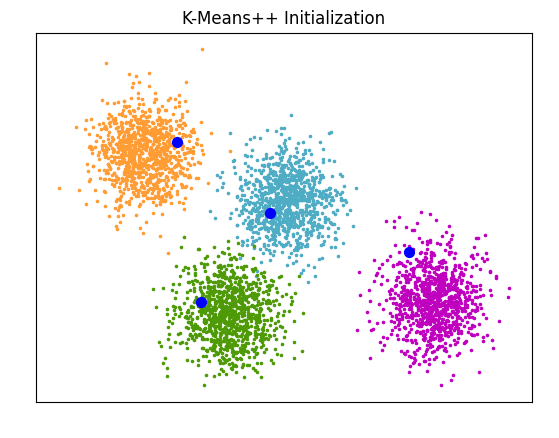3 案例1

3.1 代码

#  -*- coding: utf-8 -*-
import numpy as np
import pandas as pd
from sklearn.cluster import KMeans, MiniBatchKMeans

def main():
# 读取数据文件
file = file.dropna()  # 删除含有缺失值的数据
# print(file.dtypes)  # 查看 df 各列的数据类型
# print(file.shape)  # 查看 df 的行数和列数

# 数据准备
z_scaler = lambda x:(x-np.mean(x))/np.std(x)  # 定义数据标准化函数
dfScaler = file[['D1','D2','D3','D4','D5','D6','D7','D8','D9','D10']].apply(z_scaler)  # 数据归一化
dfData = pd.concat([file[['地区']], dfScaler], axis=1)  # 列级别合并
df = dfData.loc[:,['D1','D2','D3','D4','D5','D6','D7','D8','D9','D10']]  # 基于全部 10个特征聚类分析
# df = dfData.loc[:,['D1','D2','D7','D8','D9','D10']]  # 降维后选取 6个特征聚类分析
X = np.array(df)  # 准备 sklearn.cluster.KMeans 模型数据
print("Shape of cluster data:", X.shape)

# KMeans 聚类分析(sklearn.cluster.KMeans)
nCluster = 4
kmCluster = KMeans(n_clusters=nCluster).fit(X)  # 建立模型并进行聚类，设定 K=4
print("Cluster centers:\n", kmCluster.cluster_centers_)  # 返回每个聚类中心的坐标
print("Cluster results:\n", kmCluster.labels_)  # 返回样本集的分类结果

# 整理聚类结果(太棒啦！)
listName = dfData['地区'].tolist()  # 将 dfData 的首列 '地区' 转换为 list
dictCluster = dict(zip(listName,kmCluster.labels_))  # 将 listName 与聚类结果关联，组成字典
listCluster = [[] for k in range(nCluster)]
for v in range(0, len(dictCluster)):
k = list(dictCluster.values())[v]  # 第v个城市的分类是 k
listCluster[k].append(list(dictCluster.keys())[v])  # 将第v个城市添加到 第k类
print("\n聚类分析结果(分为{}类):".format(nCluster))  # 返回样本集的分类结果
for k in range(nCluster):
print("第 {} 类：{}".format(k, listCluster[k]))  # 显示第 k 类的结果

return

if __name__ == '__main__':
main()

3.2 结果

0  北京  5.96  310  461  1557  931  319  44.36  2615  2.20  13631
1  上海  3.39  234  308  1035  498  161  35.02  3052  0.90  12665
2  天津  2.35  157  229   713  295  109  38.40  3031  0.86   9385
3  陕西  1.35   81  111   364  150   58  30.45  2699  1.22   7881
4  辽宁  1.50   88  128   421  144   58  34.30  2808  0.54   7733
Shape of cluster data: (30, 10)
Cluster centers:
[[-3.04626787e-01 -2.89307971e-01 -2.90845727e-01 -2.88480032e-01
-2.85445404e-01 -2.85283077e-01 -6.22770669e-02  1.12938023e-03
-2.71308432e-01 -3.03408599e-01]
[ 4.44318512e+00  3.97251590e+00  4.16079449e+00  4.20994153e+00
4.61768098e+00  4.65296699e+00  2.45321197e+00  4.02147595e-01
4.22779099e+00  2.44672575e+00]
[ 1.52987871e+00  2.10479182e+00  1.97836141e+00  1.92037518e+00
1.54974999e+00  1.50344182e+00  1.13526879e+00  1.13595799e+00
8.39397483e-01  1.38149832e+00]
[ 4.17353928e-01 -6.60092295e-01 -5.55528420e-01 -5.50211065e-01
-2.95600461e-01 -2.42490616e-01 -3.10454580e+00 -2.70342746e+00
1.14743326e+00  2.67890118e+00]]
Cluster results:
[1 2 2 0 0 0 0 0 0 0 0 0 0 0 0 0 0 0 0 0 0 0 0 0 3 0 0 0 0 0]

Process finished with exit code 0

4 案例2

4.1 案例——数据

（1）数据介绍：

（2）实验目的：

1999年全国31个省份城镇居民家庭平均每人全年消费性支出数据：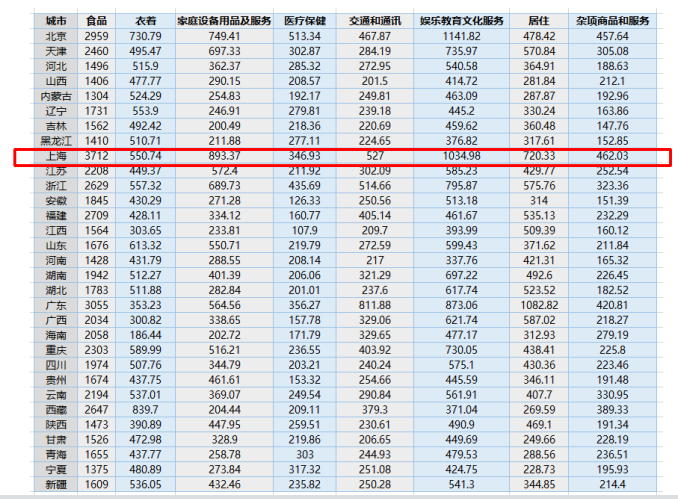4.2 代码

#*========================1. 建立工程，导入sklearn相关包======================================**

import numpy as np
from sklearn.cluster import KMeans

#*======================2. 加载数据，创建K-means算法实例，并进行训练，获得标签====================**

fr = open(filePath, 'r+')            #r+：读写打开一个文本文件
retData = []                     #retData：用来存储城市的各项消费信息
retCityName = []                 #retCityName：用来存储城市名称
for line in lines:
items = line.strip().split(",")
retCityName.append(items)
retData.append([float(items[i]) for i in range(1, len(items))])
return retData, retCityName      #返回值：返回城市名称，以及该城市的各项消费信息

def main():
km = KMeans(n_clusters=4)                #2.创建实例
label = km.fit_predict(data)             #3.调用Kmeans（）fit_predict()方法进行计算
expenses = np.sum(km.cluster_centers_, axis=1)
# print(expenses)
CityCluster = [[], [], [], []]          #将城市按label分成设定的簇
for i in range(len(cityName)):
CityCluster[label[i]].append(cityName[i])   #将每个簇的城市输出
for i in range(len(CityCluster)):              #将每个簇的平均花费输出
print("Expenses:%.2f" % expenses[i])
print(CityCluster[i])

if __name__ == '__main__':
main()

#*=============3. 输出标签，查看结果========================================**

#将城市按照消费水平n_clusters类，消费水平相近的城市聚集在一类中
#expense：聚类中心点的数值加和，也就是平均消费水平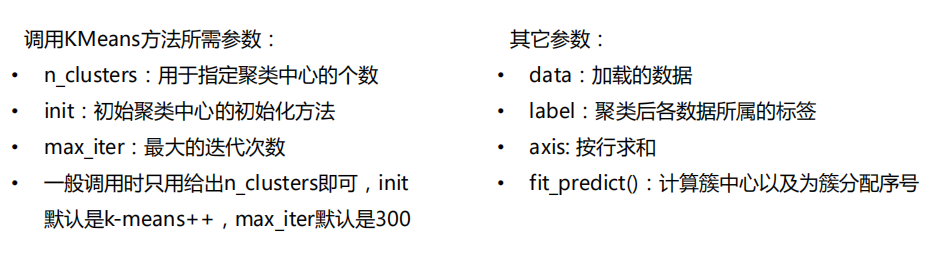4.3 结果

（1）聚成2类：km = KMeans(n_clusters=2)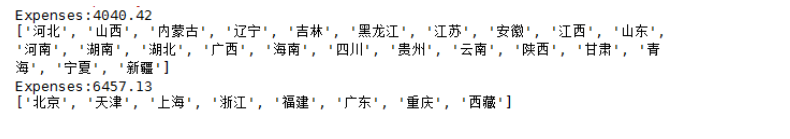（2）聚成3类：km = KMeans(n_clusters=3)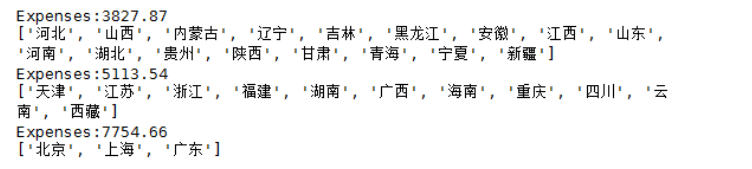（3）聚成4类：km = KMeans(n_clusters=4)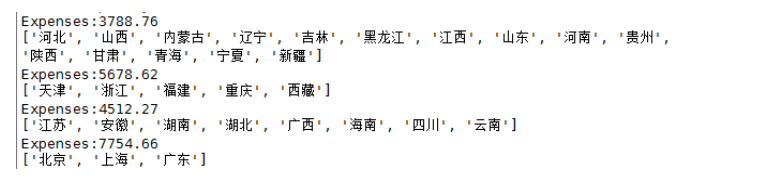4.4 拓展&&改进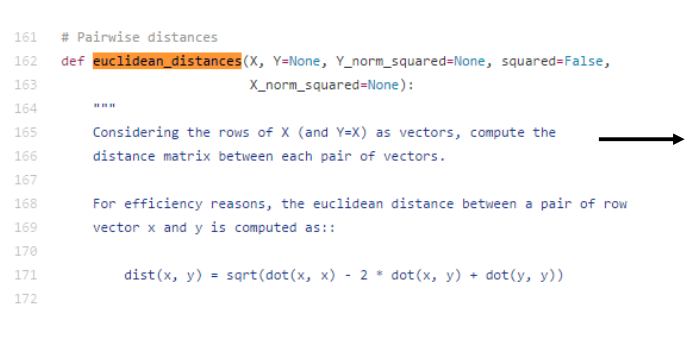（1）如果想要自定义计算距离的方式时，可以更改此处源码。
（2）建议使用 scipy.spatial.distance.cdist 方法。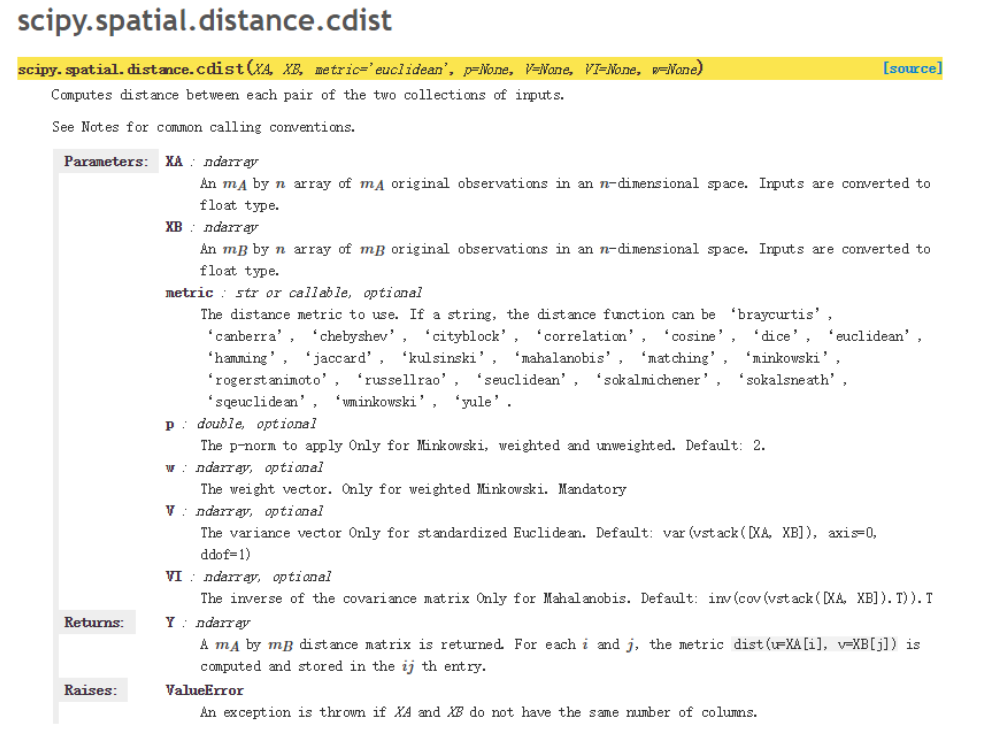• A：A向量
• B：B向量
• metric: 计算A和B距离的方法，更改此参

利用Python如何实现K-means聚类算法Python实现K-means聚类算法并可视化生成动图步骤详解K-means算法介绍 简单来说,K-means算法是一种无监督算法,不需要事先对数据集打上标签,即ground-truth,也可以对数据集进行分类,并且可以指定类别数目 牧师-村民模型 K-means 有一个著名的解释:牧师-村民模型: 有四个牧师去郊区布道,一开始牧师们随意选了几个布道点,并且把这几个布道点的情况公告给了郊区所有的村民,于是每个村民到离自己家最近的布道点去听课. 听课之后,大家觉得距离太远了,于是每个牧师统计了一下自己的课上所有的村民的地址,搬到了所有地址的中心地带,并且在海

python中实现k-means聚类算法详解Python实现的KMeans聚类算法实例分析K均值聚类算法的Java版实现代码示例

1.简介 K均值聚类算法是先随机选取K个对象作为初始的聚类中心.然后计算每个对象与各个种子聚类中心之间的距离,把每个对象分配给距离它最近的聚类中心.聚类中心以及分配给它们的对象就代表一个聚类.一旦全部对象都被分配了,每个聚类的聚类中心会根据聚类中现有的对象被重新计算.这个过程将不断重复直到满足某个终止条件.终止条件可以是没有(或最小数目)对象被重新分配给不同的聚类,没有(或最小数目)聚类中心再发生变化,误差平方和局部最小. 2.什么是聚类 聚类是一个将数据集中在某些方面相似的数据成员进行分类组织

python实现聚类算法原理Python机器学习之K-Means聚类实现详解